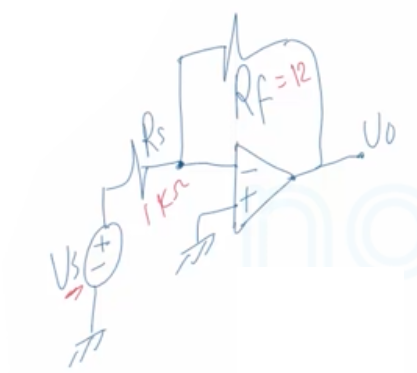Need Help?

Subscribe to Circuit

###### \${selected_topic_name}
• Notes
• Comments & Questions

$\begin{array}{l}{\text { a) Design an inverting amplifier with a gain of } 12 . \text { Use } \pm 15 \mathrm{V} \text { power supplies and an ideal op amp. }} \\ {\text { b) What range of input voltages, } v_{s}, \text { allows the op amp in this design to remain in its linear Region? }}\end{array}$$Gain=12=\frac{R_ f}{R_ s}$

$R_{s}=1k \Omega \Rightarrow R_f=12R_s=12k \Omega$

$-V_{c c} \leq V_{0} \leq V_{c c}$

$\text { Inverting Amplifier }$

$V_{0}=-\text { Gain } V_{s}$

$V_{0}=-12 \mathrm{Vs}$

$-V_{c c} \leq-12 V _s \leq V_{ c c}$

$\frac{-15}{-12} \leq \frac{-12V_{s}}{-12} \leq \frac{15}{-12}$

$1.25 \geq V_s \geq -1.25$

No comments yet